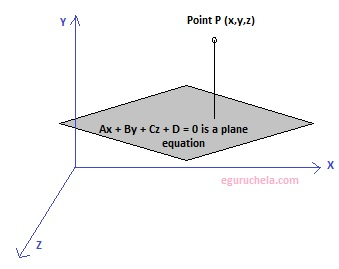# Shortest Distance Between Point and Plane Calculator

Calculates the shortest distance in space between given point and plane equation.

#### Enter the equation of the plane :

 x + y + z + = 0

 Shortest distance between point and plane

The distance from a point to a plane is equal to length of the perpendicular lowered from a point on a plane.

If Ax + By + Cz + D = 0 is a plane equation, then distance from point P(Px , Py , Pz) to plane can be found using the following formula:

The distance from a point to a plane(d) = (APx + BPy + CPz + D)/ √(A+ B2 + C2)